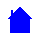## Full Subtractor Fully Explained

Let us subtract 1581 from 3398 using 12 full-subtractors

The subtractors are labelled A thru L in the diagram HERE

and the truth table is shown HERE

Notice that each row of the Truth Table corresponds to one (or more) of the subtractors..... this example was carefully designed by me to include all possible conditions that could occur in a subtractor.EL10B Homepage